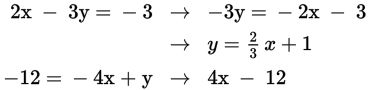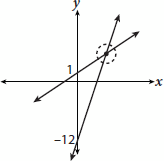# SAT Math Multiple Choice Question 802: Answer and Explanation

### Test Information

Question: 802

2x – 3y = –3

–12 = –4x + y

7. In what quadrant will the lines represented by the equations above intersect?

Explanation:

A

Difficulty: Medium

Category: Heart of Algebra / Systems of Linear Equations

Strategic Advice: Whenever you're only asked to identify the quadrant in which the graphs of two lines intersect (not the exact values of x and y), drawing a quick sketch of the system of equations is likely to be the most time-efficient method to answer the question.

Getting to the Answer: Start by writing each equation in slope-intercept form (y = mx + b):Then plot each y-intercept and use the corresponding slope to rise (or fall) and run to the next point. You don't have to be exact-no need to waste time drawing tick marks or labeling the graph-a sketch is all you need. (You can also graph the lines in your calculator if you prefer.) Your graph might look something like this:The lines intersect in quadrant I, which is (A).